# Solution assignment 03 Logarithmic functions and graphs

### Assignment 3

Sketch the graphs of: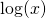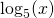### Solution

The graphs of these three logarithmic functions go through the point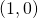and are defined for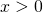. We notice that the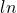function (in the graph denoted as) increases the fastest whenincreases, the logarithm with base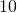the least fast. The logarithm wit base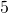is just in between. Give an explanation for this.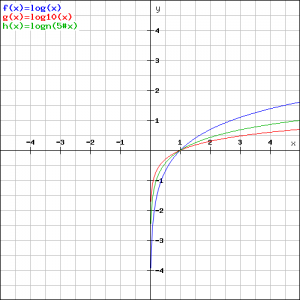0